Most Affordable JEE | NEET | 8,9,10 Preparation by Kota's Top IITian Doctor Faculties

# Electrostatics - JEE Advanced Previous Year Questions with Solutions`
JEE Advanced Previous Year Questions of Physics with Solutions are available at eSaral. Practicing JEE Advanced Previous Year Papers Questions of Physics will help the JEE aspirants in realizing the question pattern as well as help in analyzing weak & strong areas. Get detailed Class 11th & 12th Physics Notes to prepare for Boards as well as competitive exams like IIT JEE, NEET etc. eSaral helps the students in clearing and understanding each topic in a better way. eSaral is providing complete chapter-wise notes of Class 11th and 12th both for all subjects.   Download eSaral app  for free study material and video tutorials. Simulator Previous Years JEE Advanced Questions
Q. A disk of radius a / 4 having a uniformly distributed charge 6C is placed in the x-y plane with its centre at (–a /2, 0, 0). A rod of length a carrying a uniformly distributed charge 8 C is placed on the x-axis from x = a/4 to x = 5a/4. Two point charges –7 C and 3 C are placed at (a/4, –a/4, 0) and (–3a/4, 3a/4, 0), respectively. Consider a cubical surface formed by six surfaces x = $\pm$ a/2, y = $\pm$ a/2, z = $\pm$ a/2. The electric flux through this cubical surface is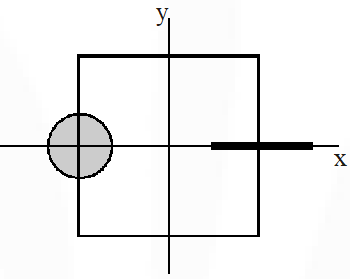$(\mathrm{A}) \frac{-2 C}{\varepsilon_{o}}$ $(\mathrm{B}) \frac{2 C}{\varepsilon_{o}}$ $(\mathrm{C}) \frac{10 C}{\varepsilon_{o}}$ $(\mathrm{D}) \frac{12 C}{\varepsilon_{o}}$ [IIT-JEE 2009]
Ans. (A)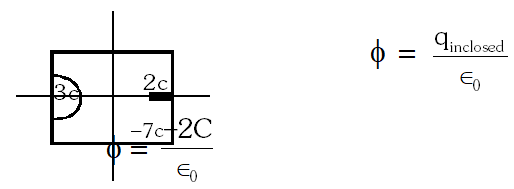Q. Three concentric metallic spherical shells of radii R, 2R, 3R, are given charges $\mathrm{Q}_{1}: \mathrm{Q}_{2}: \mathrm{Q}_{3}$ respectively. It is found that the surface charge densities on the outer surfaces of the shells are equal. Then, the ratio of the charges given to the shells, $\mathrm{Q}_{1}: \mathrm{Q}_{2}: \mathrm{Q}_{3}$ is – (A) 1 : 2 : 3 (B) 1 : 3 : 5 (C) 1 : 4 : 9 (D) 1 : 8 : 18 [IIT-JEE 2009]
Ans. (B)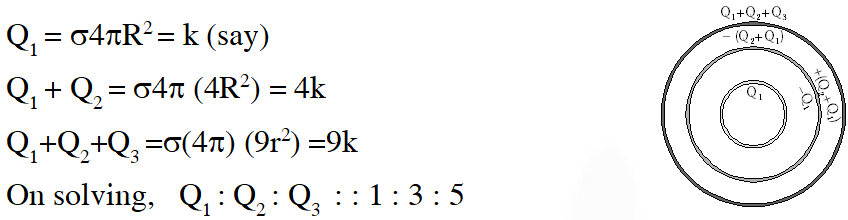Q. Under the influence of the Coulomb field of charge +Q, a charge –q is moving around it in an elliptical orbit. Find out the correct statement(s). (A) The angular momentum of the charge –q is constant (B) The linear momentum of the charge –q is constant (C) The angular velocity of the charge –q is constant (D) The linear speed of the charge –q is constant [IIT-JEE 2009]
Ans. (A) Since torque about central charge is zero angular momentum is conserved.
Q. A solid sphere of radius R has a charge Q distributed in its volume with a charge density  = $K r^{\mathrm{a}}$, where K and a are constants and r is the distance from its centre. If the electric field at $r=\frac{R}{2}$ is $\frac{1}{8}$ times that at r = R, find the value of a. [IIT-JEE 2009]
Ans. 2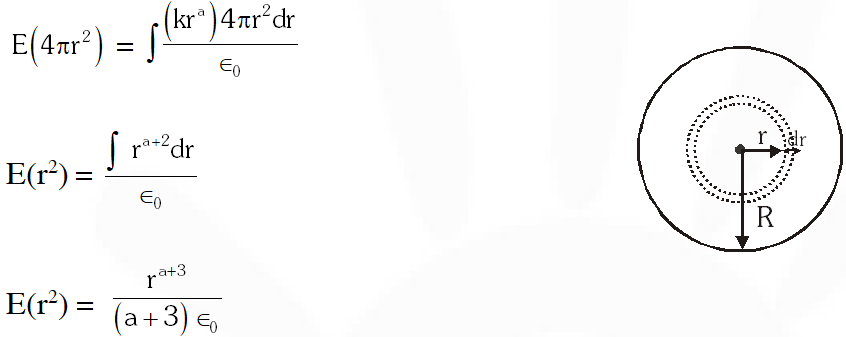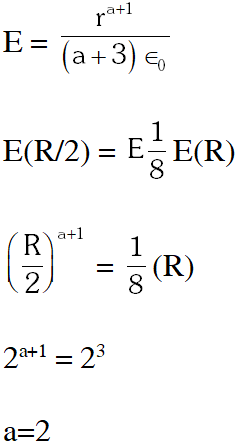Q. A uniformly charged thin spherical shell of radius R carries uniform surface charge density of $\sigma$ per unit area. It is made of two hemispherical shells, held together by pressing them with force F (see figure). F is proportional to –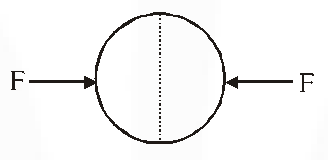(A) $\frac{1}{\varepsilon_{0}} \sigma^{2} R^{2}$ (B) $\frac{1}{\varepsilon_{0}} \sigma^{2} R$ (C) $\frac{1}{\varepsilon_{0}} \frac{\sigma^{2}}{R}$ $(\mathrm{D}) \frac{1}{\varepsilon_{0}} \frac{\sigma^{2}}{R^{2}}$ [IIT-JEE 2010]
Ans. (A) Electrostatic pressure $\mathrm{P}=\frac{\sigma^{2}}{2 \epsilon_{0}} \Rightarrow \mathrm{F}=\frac{\sigma^{2}}{2 \epsilon_{0}} \cdot \pi \mathrm{R}^{2} \Rightarrow \mathrm{F} \propto \frac{\sigma^{2} \mathrm{R}^{2}}{\epsilon_{0}}$
Q. A tiny spherical oil drop carrying a net charge q is balanced in still air with a vertical uniform electric field of strength $\frac{81 \pi}{7} \times 10^{5} \mathrm{Vm}^{-1}$. When the field is switched off, the drop is observed to fall with terminal velocity $2 \times 10^{-3} \mathrm{m} / \mathrm{s} .$ Given $g=9.8 \mathrm{m} / \mathrm{s}^{2}$, viscosity of the air = $1.8 \times 10^{-5} \mathrm{N}-\mathrm{s} \mathrm{m}^{-2}$ and the density of oil = $900 \mathrm{kg} \mathrm{m}^{-3}$, the magnitude of q is – (A) $1.6 \times 10^{-19} \mathrm{C}$ (B) $3.2 \times 10^{-19} \mathrm{C}$ (C) $4.8 \times 10^{-19} \mathrm{C}$ (D) $8.0 \times 10^{-19} \mathrm{C}$ [IIT-JEE 2010]
Ans. (D) $\mathrm{qE}=\mathrm{mg} \ldots(\mathrm{i}) ; \mathrm{mg}=6 \mathrm{phrV} \ldots(\mathrm{ii})$ and $\mathrm{m}=\frac{4}{3} \pi \mathrm{r}^{3} \mathrm{gp} \ldots(\mathrm{iii})$ On solving above equations we get $q=8.0 \times 10^{-19} \mathrm{C}$
Q. A few electric field lines for a system of two charges $Q_{1}$ and $Q_{2}$ fixed at two different points on the x-axis are shown in the figure. These lines suggest that –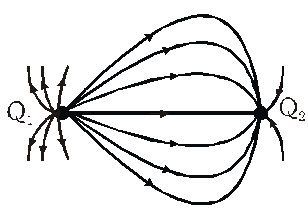(A) $\left|Q_{1}\right|>\left|Q_{2}\right|$ (B) $\left|Q_{1}\right|<\left|Q_{2}\right|$ (C) at a finite distance to the left of $Q_{1}$ the electric field is zero (D) at a finite distance to the right of $Q_{2}$ the electric field is zero [IIT-JEE 2010]
Ans. (A,D) Number of lines of force exiting from $\mathrm{Q}_{1}$ is greater than that of enetering in $\mathrm{Q}_{2} \Rightarrow\left|\mathrm{Q}_{1}\right|>\left|\mathrm{Q}_{2}\right|$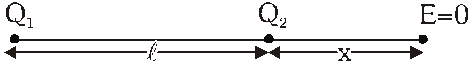$\frac{\mathrm{KQ}_{1}}{(\ell+\mathrm{x})^{2}}=\frac{\mathrm{KQ}_{2}}{\left(\mathrm{x}^{2}\right)} \Rightarrow \frac{\mathrm{x}}{(\ell+\mathrm{x})}=\sqrt{\frac{\mathrm{Q}_{1}}{\mathrm{Q}_{2}}}$ because $\mathrm{x}>0$ so there will be point in right of $\mathrm{Q}_{2}$
Q. Consider an electric field $\vec{E}=E_{0} \hat{x},$ where $\mathrm{E}_{0}$ E0 is a constant. The flux through the shaded area (as shown in the figure) due to this field is –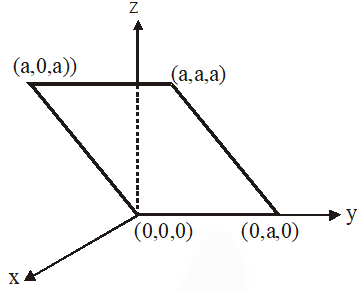(A) $2 E_{0} a^{2}$ (B) $\sqrt{2} E_{0} a^{2}$ $(\mathrm{C}) E_{0} a^{2}$ (D) $\frac{E_{0} a^{2}}{\sqrt{2}}$ [IIT-JEE 2011]
Ans. (C) $\phi=\int \bar{E} \cdot \overrightarrow{d S}=$ Ex projected area perpendiuclar to E $(\mathrm{x}-\mathrm{axis})=\mathrm{E} \times \mathrm{a}^{2}$
Q. A spherical metal shell A of radius $R_{A}$ and a solid metal sphere B of radius $R_{B}\left( (A)$E_{A}^{\text {inside }}=0$(B)$Q_{A}>Q_{B}$(C)$\frac{\sigma_{A}}{\sigma_{B}}=\frac{R_{B}}{R_{A}}$(D)$E_{A}^{\text {on sufface }} [IIT-JEE 2011]
Ans. (A,B,C,D)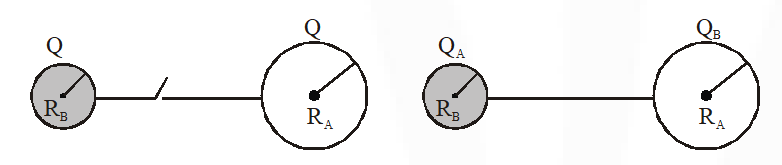$\mathrm{E}_{A}^{\text {inside }}=0$ (because of electrostatic condition) So, A option is true. $\Rightarrow \mathrm{v}_{\mathrm{A}}=\mathrm{v}_{\mathrm{B}} \Rightarrow \frac{k Q_{A}}{R_{A}}=\frac{k Q_{B}}{R_{B}} \Rightarrow \frac{Q_{A}}{Q_{B}}=\frac{R_{A}}{R_{B}} \Rightarrow \mathrm{R}_{\mathrm{B}}<\mathrm{R}_{\mathrm{A}} \mathrm{So}, \mathrm{Q}_{\mathrm{B}}<\mathrm{Q}_{\mathrm{A}},$ so $\mathrm{B}$ is true $\Rightarrow \frac{\sigma_{A} 4 \pi R_{A}^{2}}{\sigma_{B} 4 \pi R_{B}^{2}}=\frac{R_{A}}{R_{B}} \Rightarrow \frac{\sigma_{A}}{\sigma_{B}}=\frac{R_{B}}{R_{A}},$ So $\mathrm{C}$ is true $\mathrm{E}_{\text {nexsurixe }}=\sigma \times \frac{1}{R} \cdot \mathrm{So}, \mathrm{D}$ is also true
Q. A wooden block performs SHM on a frictionless surface with frequency, $v_{0}$. The block carries a charge +Q on its surface. If now a uniform electric field $\vec{E}$ is switched-on as shown, then the SHM of the block will be –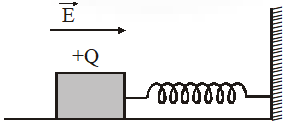(A) of the same frequency and with shifted mean position (B) of the same frequency and with the same mean position (C) of changed frequency and with shifted mean position (D) of changed frequency and with the same mean position [IIT-JEE 2011]
Ans. (A) Time period of spring block system depends on spring constant and mass of block. On applying electric field only the equilibrium position gets shifted.
Q. Which of the following statement(s) is/are correct? (A) If the electric field due to a point charge varies as $r^{-2.5}$ instead of $r^{-2}$, then the Gauss law will still be valid. (B) The Gauss law can be used to calculate the field distribution around an electric dipole. (C) If the electric field between two point charges is zero somewhere, then the sign of the two charges is the same. (D) The work done by the external force in moving a unit positive charge from point A at potential $V_{A}$ to point $\mathrm{B}$ at potential $V_{B}$ is $\left(V_{B}-V_{A}\right)$ [IIT-JEE 2011]
Ans. (C,D) The field distribution for a dipole can not be calculated by using Gauss law only, therefore (C,D)
Q. Four point charges, each of +q, are rigidly fixed at the four corners of a square planar soap film of side ‘’. The surface tension of the soap film is $\gamma$. The system of charges and planar film are in equilibrium, and $a=k\left[\frac{q^{2}}{\gamma}\right]^{1 / N}$, where ‘k’ is a constant. Then N is - [IIT-JEE 2011]
Ans. 3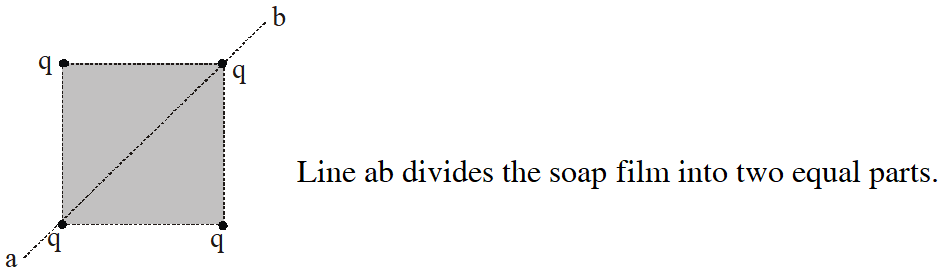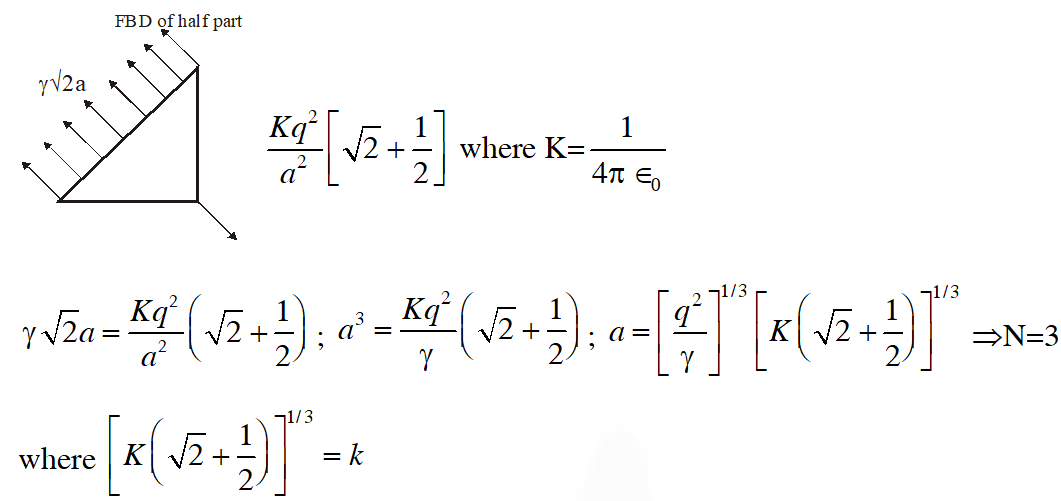Paragraph for Question Nos. 13 and 14 A dense collection of equal number of electrons and positive ions is called neutral plasma. Certain solids containing fixed positive ions surrounded by free electrons can be treated as neutral plasma. Let ‘N’ be the number density of free electrons, each of mass ‘m’. When the electrons are subjected to an electric field, they are displaced relatively away from the heavy positive ions. If the electric field becomes zero, the electrons begins to oscillate about the positive ions with a natural angular frequency ‘$\omega_{\rho}$’, which is called the plasma frequency. To sustain the oscillations, a time varying electric field needs to be applied that has an angular frequency $\omega$, where a part of the energy is absorbed and a part of it is reflected. As $\omega$ approaches $\omega_{p}$, all the free electrons are set to resonance together and all the energy is reflected. This is the explanation of high reflectivity of metals
Q. Taking the electronic charge as ‘e’ and the permittivity as ‘$\varepsilon_{0}$’, use dimensional analysis to determine the correct expression for $\omega_{\rho}$. $(\mathrm{A}) \sqrt{\frac{N e}{m \varepsilon_{0}}}$ (B) $\sqrt{\frac{m \varepsilon_{0}}{N e}}$ (C) $\sqrt{\frac{N e^{2}}{m \varepsilon_{0}}}$ $(\mathrm{D}) \sqrt{\frac{m \varepsilon_{0}}{N e^{2}}}$ [IIT-JEE 2011]
Ans. (C) Dimension of $\omega_{P}$ is $T^{-1}$ $[\sqrt{\frac{N e^{2}}{n E_{0}}}]=T^{-1}$ $2 \pi f=\omega P=\sqrt{\frac{N e^{2}}{n E_{0}}}$
Q. Estimate the wavelength at which plasma reflection will occur for a metal having the density of electrons $N \approx 4 \times 10^{27} m^{-3} .$ Take $\varepsilon_{0} \approx 10^{-11}$ and $m \approx 10^{-30}$, where these quantities are in proper SI units (A) 800 nm (B) 600 nm (C) 300 nm (D) 200 nm [IIT-JEE 2011]
Ans. (B) $\omega=\sqrt{\frac{N e^{2}}{m E_{0}}} 2 \pi f$ $\lambda=\frac{C}{f}$
Q. An infinitely long solid cylinder of radius R has a uniform volume charge density . It has a spherical cavity of radius R/2 with its centre on the axis of the cylinder, as shown in the figure. The magnitude of the electric field at the point P, which is at a distance 2R from the axis of the cylinder, is given by the expression $\frac{23 \rho R}{16 k \varepsilon_{0}}$. The value of k is -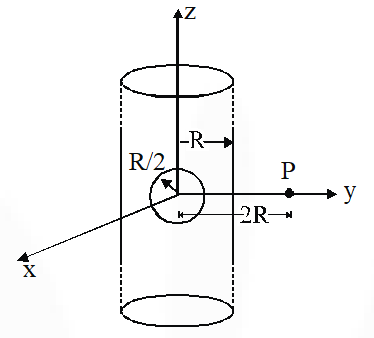[IIT-JEE 2012]
Ans. 6 Electric field due to cylinder $=\frac{2 K \lambda}{2 R}$ Electric field due to sphere $=\frac{K Q}{(2 R)^{2}}\left[\text { where } K=\frac{1}{4 \pi \epsilon_{0}}\right]$ Electric field due to sphere $=\frac{K Q}{(2 R)^{2}}\left[\text { where } K=\frac{1}{4 \pi \epsilon_{0}}\right]$ $E=\frac{2 K(\lambda)}{2 R}-\frac{K(Q)}{(2 R)^{2}}=\frac{K\left(\rho \pi R^{2}\right)}{R}-\frac{K\left(\rho \times \frac{4 \pi}{3}\left(\frac{R^{3}}{8}\right)\right)}{4 R^{2}}$ $=K(\rho \pi R)-\frac{K(\rho)(\pi R)}{24}=\frac{23 \rho \pi R}{4 \pi \varepsilon_{0} \times 24}=\frac{23 \rho R}{16 \times 6 \varepsilon_{0}} \Rightarrow k=6$
Q. A cubical region of side a has its centre at the origin. It encloses three fixed point charges, –q at (0, –a/4, 0), +3q at (0,0,0) and –q at (0, +a/4, 0). Choose the correct option(s).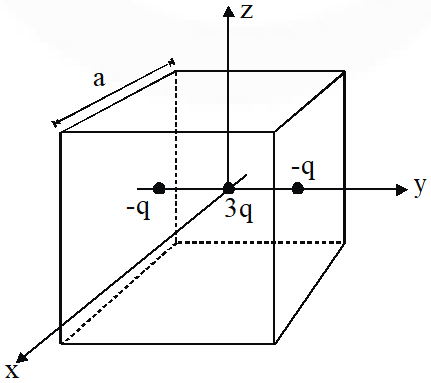(A) The net electric flux crossing the plane x = +a/2 is equal to the net electric flux crossing the plane x = – a/2 (B) The net electric flux crossing the plane y = +a/2 is more than the net electric flux crossing the plane y = –a/2. (C) The net electric flux crossing the entire region is $\frac{q}{\varepsilon_{0}}$ (D) The net electric flux crossing the plane z = +a/2 is equal to the net electric flux crossing the plane x = +a/2. [IIT-JEE 2012]
Ans. (A,C,D) For (A): Position of charges is symmetrical with respect to planes at x = + a/2 and x = – a/2 For (C) : Net charge enclosed by cube is q. For (D) : Charges are symmetrically placed iwth given planes.
Q. Two large vertical and parallel metal plates having a separation of 1 cm are connected to a DC voltage source of potential difference X. A proton is released at rest midway between the two plates. It is found to move at $45^{\circ}$ to the vertical JUST after release. Then X is nearly – (A) $1 \times 10^{-5} \mathrm{V}$ (B) $1 \times 10^{-7} \mathrm{V}$ (C) $1 \times 10^{-9} \mathrm{V}$ (D) $1 \times 10^{-10} \mathrm{V}$ [IIT-JEE 2012]
Ans. (C)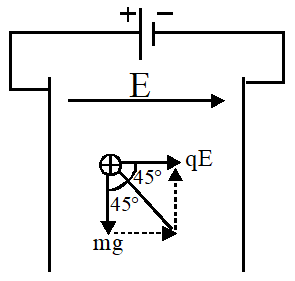As proton moves at $45^{\circ}$ in vertical plane it implies magnitude of electric force and gravitational force is same. for $45^{\circ}$ direction $|\mathrm{mg}|=|\mathrm{qE}|$ $\frac{m g}{q}=E=\frac{V}{d}$ $V=\frac{m g d}{q}=\frac{\left(1.6 \times 10^{-27} \mathrm{kg}\right)\left(1 \times 10^{-2} \mathrm{m}\right)}{\left(1.6 \times 10^{-19} \mathrm{C}\right)}=1 \times 10^{-9} \mathrm{volt}$
Q. Consider a thin spherical shell of radius R with its centre at the origin, carrying uniform positive surface charge density. The variation of the magnitude of the electric field $|\vec{E}(r)|$ and the electric potential V(r) with the distance r from the centre, is best represented by which graph ?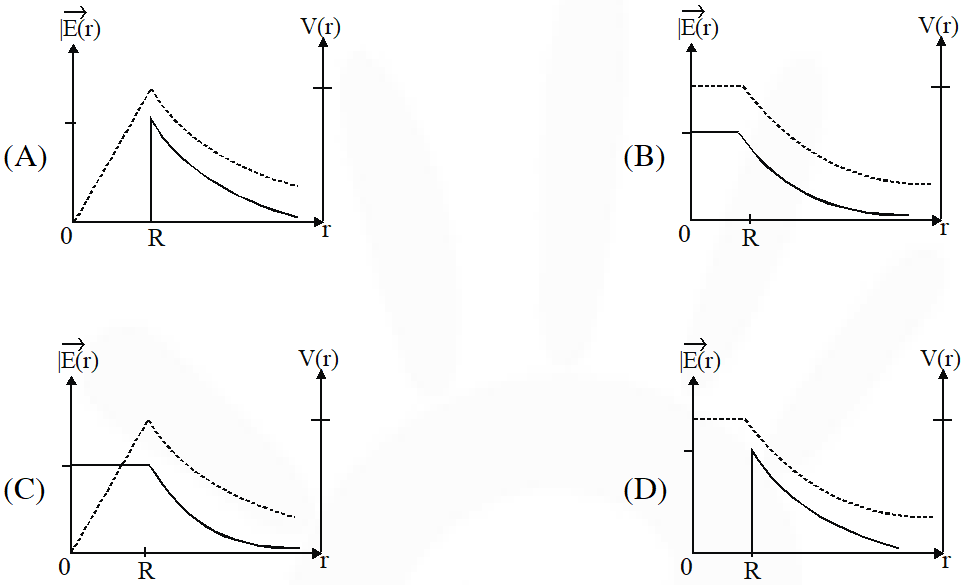[IIT-JEE 2012]
Ans. (D) Electric field inside a uniformly charged spherical shell :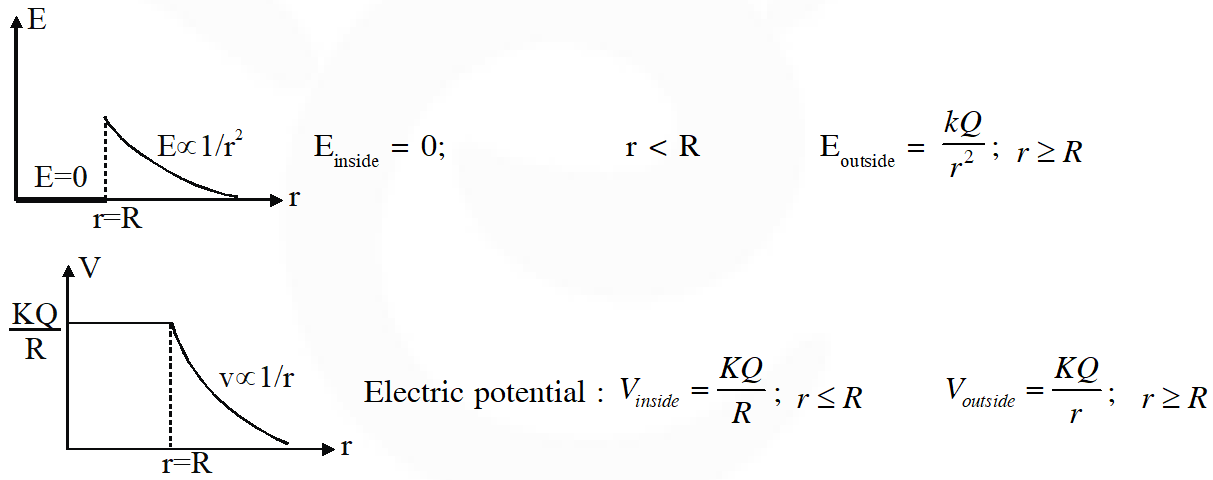Q. Six point charges are kept at the vertices of a regular hexagon of side L and centre O, as shown in figure. Given that $K=\frac{1}{4 \pi \epsilon_{0}} \frac{q}{L^{2}}$, which of the following statement(s) is(are) correct ?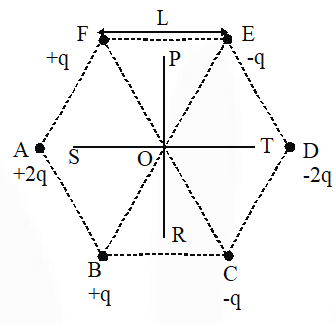(A) The electric field at O is 6 K along OD. (B) The potential at O is zero. (C) The potential at all points on the line PR is same. (D) The potential at all points on the line ST is same. [IIT-JEE 2012]
Ans. (A,B,C)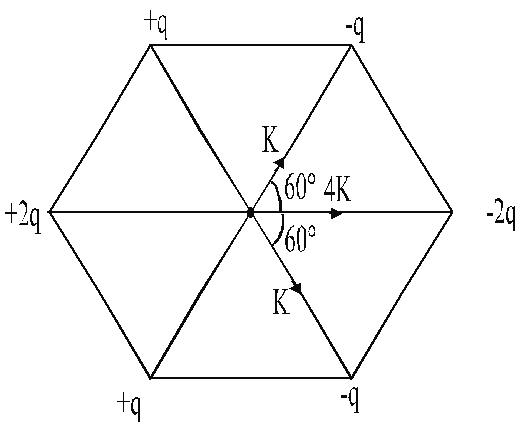Resultant is 6 K along OD. For every point of POR there are two charges of opposite sign and equal magnitude at equal distance.
Q. Two non-conducting solid spheres of radii R and 2R, having uniform volume charge densities $\rho_{1}$ and $\rho_{2}$respectively, touch each other. The net electric field at a distance 2R from the centre of the smaller sphere, along the line joining the centres of the spheres, is zero. The ratio $\frac{\rho_{1}}{\rho_{2}}$ can be- (A) $-4$ $(\mathrm{B})-\frac{32}{25}$ (C) $\frac{32}{25}$ (D) 4 [JEE-Advance-2013]
Ans. (B,D) $\mathrm{q}_{1}=\rho_{1} \frac{4}{3} \pi \mathrm{R}^{3} ; \mathrm{q}_{2}=\rho_{2} \frac{4}{3} \pi(2 \mathrm{R})^{3}$ if $\mathrm{E}_{\mathrm{net}}=0$ at point A then $\frac{\mathrm{kq}_{1}}{(2 \mathrm{R})^{2}}+\frac{\mathrm{kq}_{2}}{(5 \mathrm{R})^{2}}=0 \Rightarrow \frac{\mathrm{q}_{1}}{\mathrm{q}_{2}}=-\frac{4}{25}$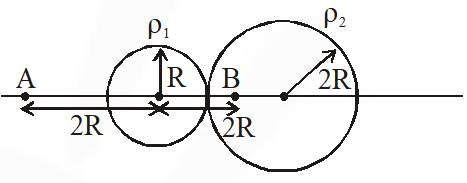$\Rightarrow \frac{\rho_{1}}{\rho_{2}}=-\frac{32}{25}$ if $\mathrm{E}_{\mathrm{net}}=0$ at point $\mathrm{B}$ then $\frac{\mathrm{kq}_{1}}{(2 \mathrm{R})^{2}}=\frac{\mathrm{k} \mathrm{q}_{2} \mathrm{R}}{(2 \mathrm{R})^{3}} \Rightarrow \frac{\mathrm{q}_{1}}{\mathrm{q}_{2}}=\frac{1}{2} \Rightarrow \frac{\rho_{1}}{\rho_{2}}=4$
Q. Two non-conducting spheres of radii $\mathrm{R}_{1}$ and $\mathrm{R}_{2}$ and carrying uniform volume charge densities + and – , respectively, are placed such that they partially overlap, as shown in the figure. At all points in the overlapping region :-(A) the electrostatic field is zero (B) the electrostatic potential is constant (C) the electrostatic field is constant in magnitude (D) the electrostatic field has same direction [JEE-Advance-2013]
Ans. (C,D) Electric field at point $P=\frac{\rho(\overline{A P})}{3 \epsilon_{0}}+\frac{-\rho(\overline{B P})}{3 \epsilon_{0}}$ $=\frac{\rho(\overline{\mathrm{AP}}-\overline{\mathrm{BP}})}{3 \epsilon_{0}}=\frac{\rho(\overline{\mathrm{AB}})}{3 \epsilon_{0}}$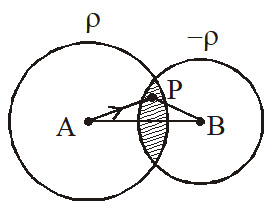= constant in both magnitude and direction at every point of overlap region. Also E is non zero so potential is not same at all points.
Q. $\mathrm{E}_{1}(\mathrm{r}), \mathrm{E}_{2}(\mathrm{r})$ and $\mathrm{E}_{3}(\mathrm{r})$ be the respective electric fields at a distance r from a point charge Q, an infinitely long wire with constant linear charge density , and an infinite plane with uniform surface charge density $\sigma .$ If $\mathrm{E}_{1}\left(\mathrm{r}_{0}\right)=\mathrm{E}_{2}\left(\mathrm{r}_{0}\right)=\mathrm{E}_{3}\left(\mathrm{r}_{0}\right)$ at a given distance $\mathrm{r}_{0}$, then :- (A) $\mathrm{Q}=4 \sigma \pi \mathrm{r}_{0}^{2}$ (B) $\mathrm{r}_{0}=\frac{\lambda}{2 \pi \sigma}$ (C) $\mathrm{E}_{1}\left(\mathrm{r}_{0} / 2\right)=2 \mathrm{E}_{2}\left(\mathrm{r}_{0} / 2\right)$ (D) $\mathrm{E}_{2}\left(\mathrm{r}_{0} / 2\right)=4 \mathrm{E}_{3}\left(\mathrm{r}_{0} / 2\right)$ [JEE-Advance-2014]
Ans. (C)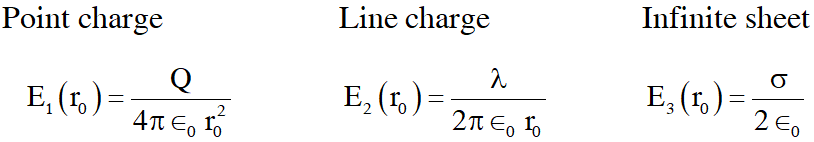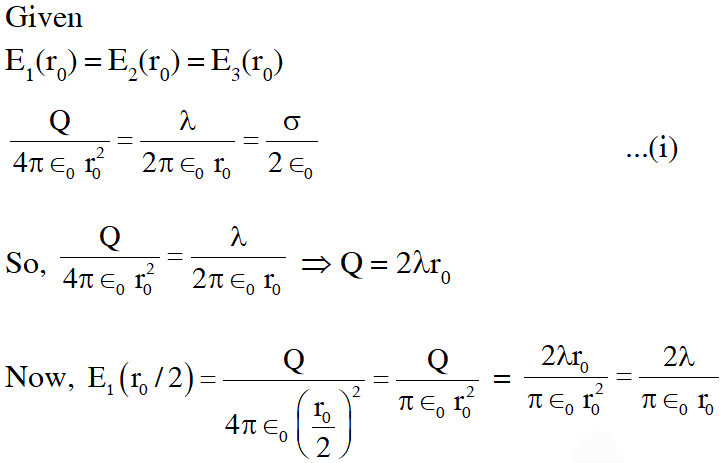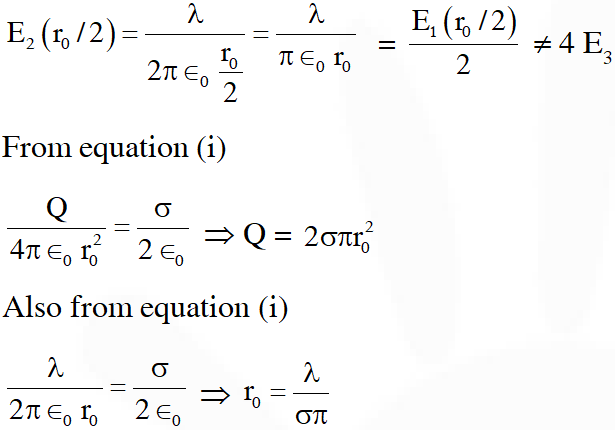Q. Charges Q, 2Q and 4Q are uniformly distributed in three dielectric solid spheres 1, 2 and 3 of radii R/2, R and 2R respectively, as shown in figure. If magnitudes of the electric fields at point P at a distance R from the centre of spheres 1, 2 and 3 are $\mathrm{E}_{1}, \mathrm{E}_{2}$ and $\mathrm{E}_{2}$respectively, then –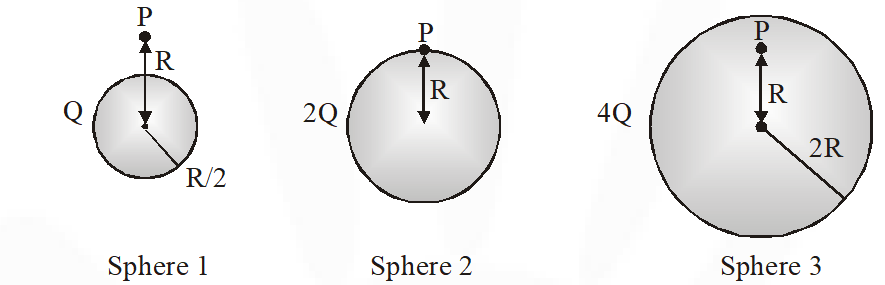$(\mathrm{A}) \mathrm{E}_{1}>\mathrm{E}_{2}>\mathrm{E}_{3}$ $(\mathrm{B}) \mathrm{E}_{3}>\mathrm{E}_{1}>\mathrm{E}_{2}$ $(\mathrm{C}) \mathrm{E}_{2}>\mathrm{E}_{1}>\mathrm{E}_{3}$ $(\mathrm{D}) \mathrm{E}_{3}>\mathrm{E}_{2}>\mathrm{E}_{1}$ [JEE-Advance-2014]
Ans. (C)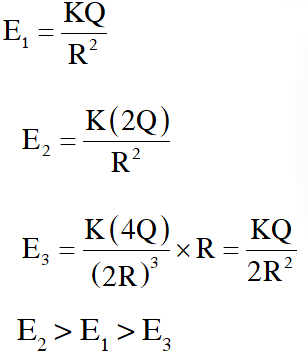Q. Four charges $\mathrm{Q}_{1}, \mathrm{Q}_{2}, \mathrm{Q}_{3}$ and $\mathrm{Q}_{4}$ of same magnitude are fixed along the x axis at x = –2a, –a, +a and +2a, respectively. A positive charge q is placed on the positive y axis at a distance b > 0. Four options of the signs of these charges are given in List I. The direction of the forces on the charge q is given in List II. Match List I with List II and select the correct answer using the code given below the lists.(A) P-3, Q-1, R-4, S-2 (B) P-4, Q-2, R-3, S-1 (C) P-3, Q-1, R-2, S-4 (D) P-4, Q-2, R-1, S-3 [JEE-Advance-2014]
Ans. (A) When all are positive net field is along +y axis $\mathrm{P} \rightarrow 3$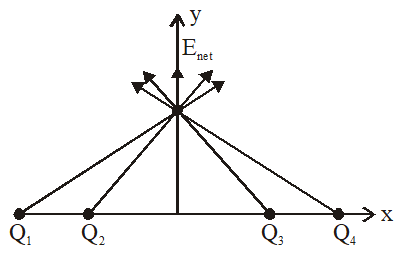when $\mathrm{Q}_{1}, \mathrm{Q}_{2}$ positive $\mathrm{Q}_{3}, \mathrm{Q}_{4}$ negative $\mathrm{Q} \rightarrow 1$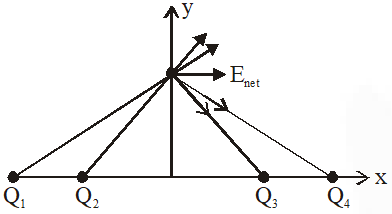When $\mathrm{Q}_{1}, \mathrm{Q}_{4}$ positive and $\mathrm{Q}_{2}, \mathrm{Q}_{3}$ negative $\mathrm{R} \rightarrow 4$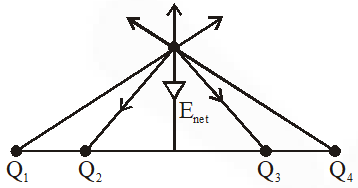Q. An infinitely long uniform line charge distribution of charge per unit length $\lambda$ lies parallel to the y-axis in the y-z plane at z = $\frac{\sqrt{3}}{2} \mathrm{a}$ (see figure). If the magnitude of the flux of the electric field through the rectangular surface ABCD lying in the x-y plane with its centre at the origin is $\frac{\lambda \mathrm{L}}{\mathrm{n} \varepsilon_{0}}$ ($\varepsilon_{0}$= perimittivity of free space) then the value of n is.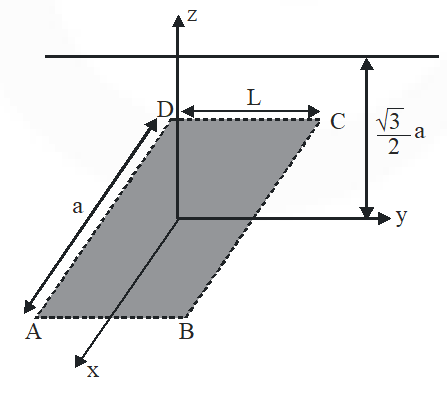[JEE-Advance-2015]
Ans. 6 Every point on circumference of flat surface is at equal distance from point charge  Hence circumference is equipotential. Flux passing through curved surface = – flux passing through flat surface.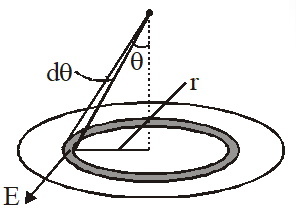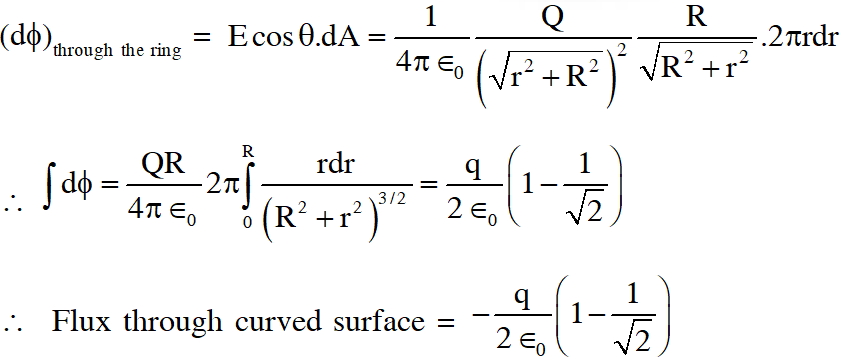Note : Flux through surface can be calculated using concept of solid angle.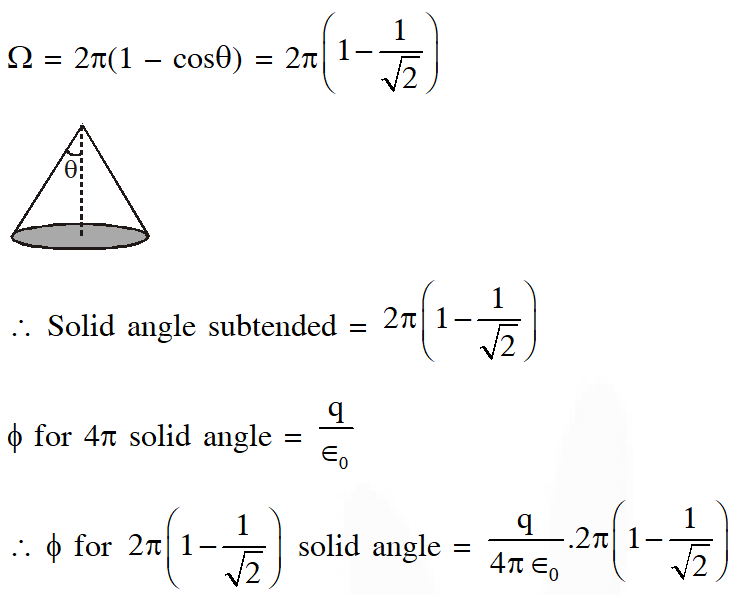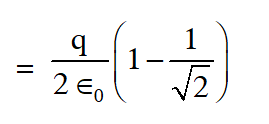Q. The figures below depict two situations in which two infinitely long static line charges of constant positive line charge density $\lambda$ are kept parallel to each other. In their resulting electric field, point charges q and –q are kept in equilibrium between them .The point charges are confined to move in the x direction only. If they are given a small displacement about their equlibrium positions, then the correct statement(s) is (are) :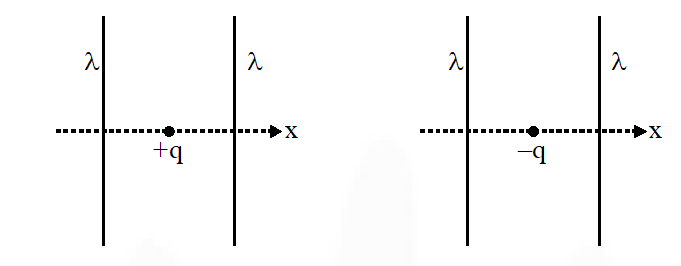(A) Both charges execute simple harmonic motion (B) Both charges will continue moving in the direction of their displacement (C) Charge +q executes simple harmonic motion while charge –q continues moving in the direction of its displacement. (D) Charge –q executes simple harmonic motion while charge +q continues moving in the direction of its displacement. [JEE-Advance-2015]
Ans. (C)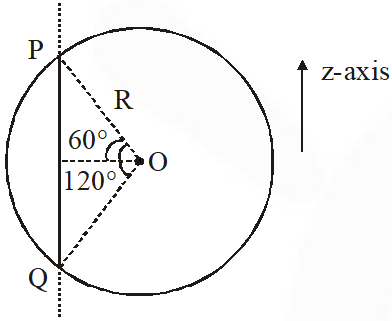Field due to straight wire is perpendicular to the wire & radially outward. Hence $\mathrm{E}_{\mathrm{z}}=0$ Length, PQ = 2R sin 60 = $\sqrt{3} \mathrm{R}$ According to Gauss's law total flux $=\left[\int \overrightarrow{\mathrm{E}} \cdot \overrightarrow{\mathrm{ds}}=\frac{q_{\mathrm{in}}}{\epsilon_{0}}=\frac{\lambda \sqrt{3} \mathrm{R}}{\epsilon_{0}}\right.$
Q. Consider a uniform spherical distirbution of radius R1 centred at the origin O. In this distibution, a spherical cavity of radius R2, centred at P with distance OP = a = $\mathrm{R}_{1}-\mathrm{R}_{2}$ (see figure) is made. If the electric field inside the cavity at position $\overrightarrow{\mathrm{r}}$ is $\overrightarrow{\mathrm{E}}(\overrightarrow{\mathrm{r}})$ , then the correct statement(s) is(are) :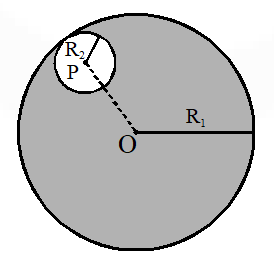(A) $\overrightarrow{\mathrm{E}}$ is uniform, its magnitude is independent of $\mathrm{R}_{2}$ but its direction depends on $\overrightarrow{\mathrm{r}}$ (B) $\overrightarrow{\mathrm{E}}$ is uniform, its magnitude depends on $\mathrm{R}_{2}$ and its direction depends on $\overrightarrow{\mathrm{r}}$ (C) $\overrightarrow{\mathrm{E}}$ is uniform, its magnitude is independent of a but its direction depends on $\vec{a}$ (D) $\overrightarrow{\mathrm{E}}$ is uniform and both its magnitude and direction depend on $\vec{a}$ [JEE-Advance-2015]
Ans. (D)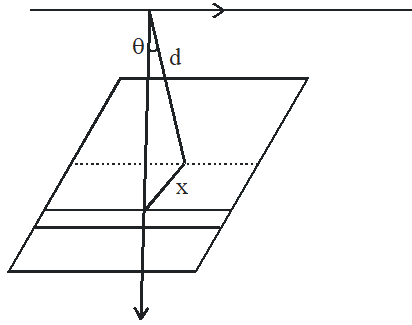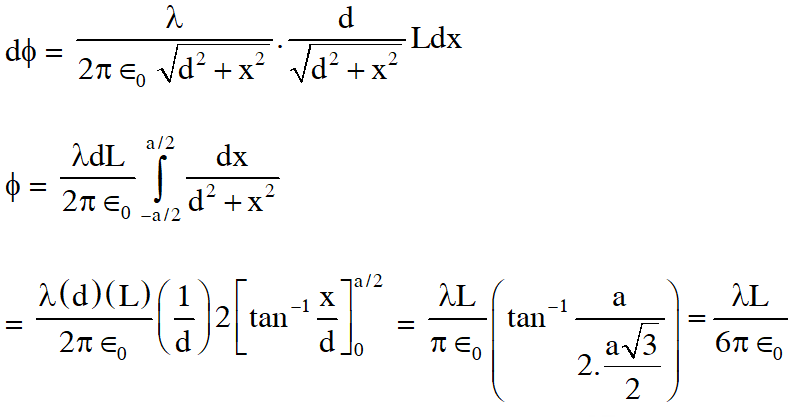Q. A length-scale $(\ell)$ depends on the permittivity $(\varepsilon)$ of a dielectric material, Boltzmann constant kB, the absolute temperature T, the number per unit volume (n) of certain charged particles, and the charge (q) carried by each of the particles, Which of the following expressions(s) for $\ell$ is(are) dimensionally correct? $(\mathrm{A}) \ell=\sqrt{\left(\frac{\mathrm{nq}^{2}}{\varepsilon \mathrm{k}_{\mathrm{B}} \mathrm{T}}\right)}$ $(\mathrm{B})^{\ell}=\sqrt{\left(\frac{\varepsilon \mathrm{k}_{\mathrm{B}} \mathrm{T}}{\mathrm{nq}^{2}}\right)}$ (C) $\ell=\sqrt{\left(\frac{\mathrm{q}^{2}}{\mathrm{n}^{2 / 3} \mathrm{k}_{\mathrm{B}} \mathrm{T}}\right)}$ (D) $\ell=\sqrt{\left(\frac{\mathrm{q}^{2}}{\varepsilon \mathrm{n}^{1 / 3} \mathrm{k}_{\mathrm{B}} \mathrm{T}}\right)}$ [JEE-Advance-2016]
Ans. (B,D) In first case if q is shifted towards one of the wires, its repulsive force will increase which will bring the charge back. In second case, in same type of displacement attractive force due to wire increases which will make it move further towards wire. $\therefore$ (C)
Q. A point charge +Q is placed just outside an imaginary hemispherical surface of radius R as shown in the figure. Which of the following statements is/are correct ?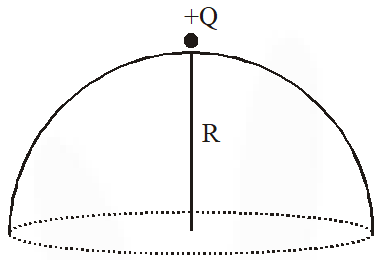(A) The circumference of the flat surface is an equipotential (B) The electric flux passing through the curved surface of the hemisphere is $-\frac{\mathrm{Q}}{2 \varepsilon_{0}}\left(1-\frac{1}{\sqrt{2}}\right)$ (C) Total flux through the curved and the flat surfaces is $\frac{\mathrm{Q}}{\varepsilon_{0}}$ (D) The component of the electric field normal to the flat surface is constant over the surface. [JEE-Advance-2017]
Ans. (A,B)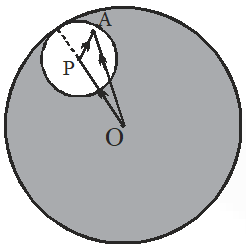Considering any point A insde the cavity electric field can be calculated at A by using super position priciple. $\overrightarrow{\mathrm{E}}_{\mathrm{A}}=\left(\overrightarrow{\mathrm{E}}_{\mathrm{A}}\right)_{\mathrm{Due} \text { to sphere without cavity }}-\left(\overrightarrow{\mathrm{E}}_{\mathrm{A}}\right)_{\text {Due to sphere of radius } \mathrm{R}_{2} \& \text { centre at } \mathrm{P}}$ $\overrightarrow{\mathrm{E}}_{\mathrm{A}}=\frac{\rho}{3 \epsilon_{0}} \mathrm{O} \overrightarrow{\mathrm{A}}=\frac{\rho}{3 \epsilon_{0}}(\mathrm{P} \overrightarrow{\mathrm{A}})$ But $\mathrm{O} \overrightarrow{\mathrm{P}}+\mathrm{P} \overrightarrow{\mathrm{A}}=\mathrm{O} \overrightarrow{\mathrm{P}}$ $\therefore \mathrm{O} \overrightarrow{\mathrm{A}}-\mathrm{P} \overrightarrow{\mathrm{A}}=\mathrm{O} \overrightarrow{\mathrm{P}}$ $\therefore \overrightarrow{\mathrm{E}}_{\mathrm{A}}=\frac{\rho}{3 \epsilon_{0}}(\mathrm{O} \overrightarrow{\mathrm{P}})=\frac{\rho}{3 \epsilon_{0}}(\overrightarrow{\mathrm{a}})$ So E.F. is independent of location of A inside the cavity. Ans. (D) is correct
Q. An infinitely long thin non-conducting wire is parallel to the z-axis and carries a uniform line charge density $\lambda$. It pierces a thin non-conducting spherical shell of radius R in such a way that the arc PQ subtends an angle $120^{\circ}$ at the centre O of the spherical shell, as shown in the figure. The permittivity of free space is $\varepsilon_{0}$. Which of the following statements is (are) true ?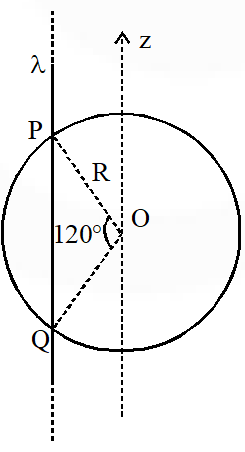(A) The electric flux through the shell is $\sqrt{3} \mathrm{R} \lambda / \varepsilon_{0}$ (B) The z-component of the electric field is zero at all the points on the surface of the shell (C) The electric flux through the shell is $\sqrt{2} \mathrm{R} \lambda / \varepsilon_{0}$ (D) The electric field is normal to the surface of the shell at all points [JEE-Advance-2018]
Ans. (A,B) We know,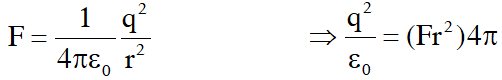So, dimension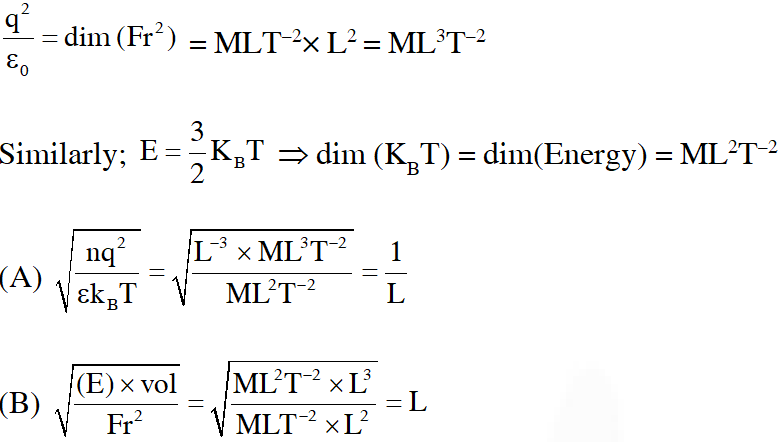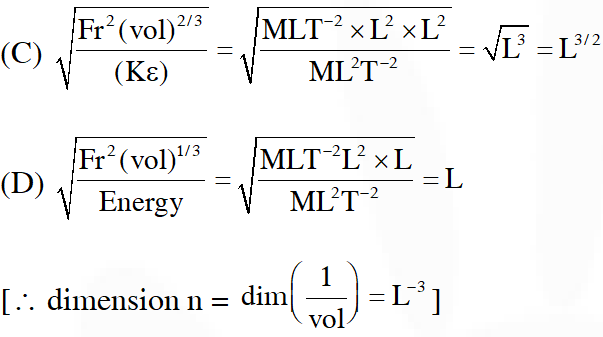Q. A particle, of mass $10^{-3}$ kg and charge 1.0 C, is initially at rest. At time t = 0, the particle comes under the influence of an electric field $\overrightarrow{\mathrm{E}}(\mathrm{t})=\mathrm{E}_{0} \sin \omega \mathrm{t} \hat{\mathrm{i}}$ where $\mathrm{E}_{0}=1.0 \mathrm{N} \mathrm{C}^{-1}$ and $\omega=10^{3}$rad $s^{-1}$. Consider the effect of only the electrical force on the particle. Then the maximum speed, in $\mathrm{ms}^{-1}$, attained by the particle at subsequent times is......................... [JEE-Advance-2018]
Ans. 2 $\mathrm{n}=10^{-3} \mathrm{kg} \mathrm{q}=1 \mathrm{C} \mathrm{t}=0$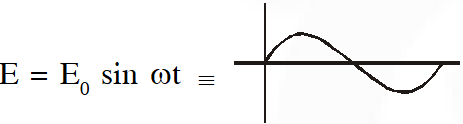Force on particle will be $\mathrm{F}=\mathrm{q} \mathrm{E}=\mathrm{q} \mathrm{E}_{0} \sin \omega \mathrm{t}$ at $\mathrm{v}_{\max }, \mathrm{a}, \mathrm{F}=0$ $\mathrm{qE}_{0} \sin \omega \mathrm{t}=0$ $\mathrm{F}=\mathrm{qE}_{0} \sin \omega \mathrm{t}$ $\frac{\mathrm{d} \mathrm{v}}{\mathrm{dt}}=\mathrm{q} \frac{\mathrm{E}_{0}}{\mathrm{m}} \sin \omega \mathrm{t}$ $\int_{0}^{\mathrm{v}} \mathrm{d} \mathrm{v}=\int_{0}^{\pi / \omega} \frac{\mathrm{q} \mathrm{E}_{0}}{\mathrm{m}} \sin \omega \mathrm{t} \mathrm{dt}$ $\mathrm{v}-0=\frac{\mathrm{q} \mathrm{E}_{0}}{\mathrm{m} \omega}[-\cos \omega \mathrm{t}]_{0}^{\pi / \omega}$ $\mathrm{v}-0=\frac{\mathrm{qE}_{0}}{\mathrm{m} \omega}[(-\cos \pi)-(-\cos 0)]$ $\mathrm{v}=\frac{1 \times 1}{10^{-3} 10^{3}} \times 2=2 \mathrm{m} / \mathrm{s}$ Ans. 2. 00
Q. The electric field E is measured at a point P(0,0,d) generated due to various charge distributions and the dependence of E on d is found to be different for different charge distributions. List-I contains different relations between E and d. List-II describes different electric charge distributions, along with their locations. Match the functions in List-I with the related charge distributions in List-II.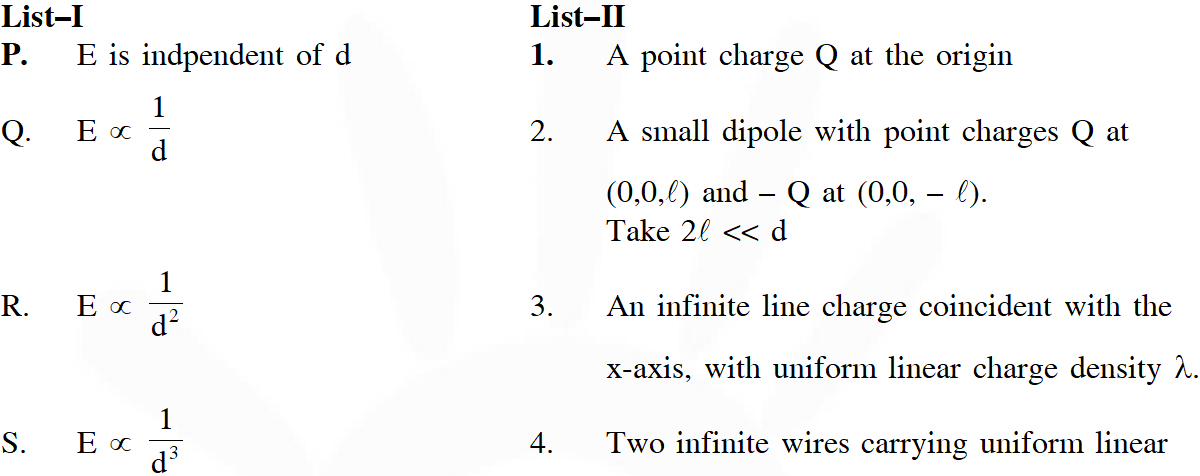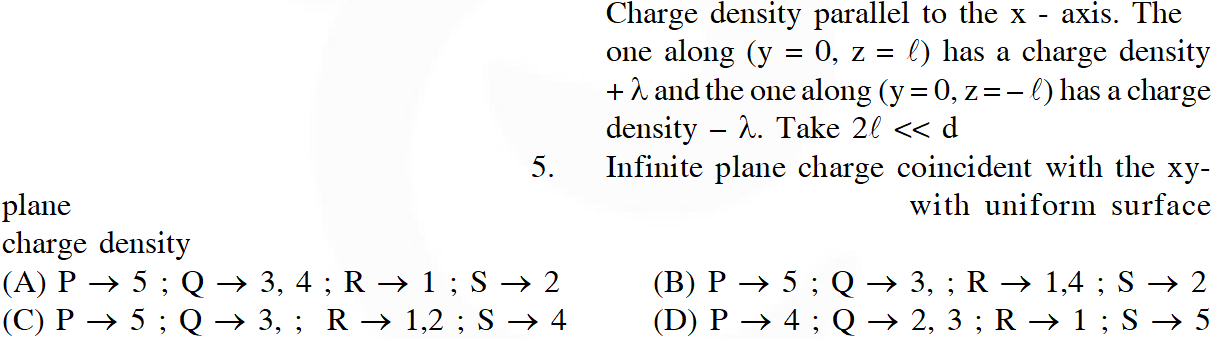[JEE-Advance-2018]
Ans. (B) (i) $\mathrm{E}=\frac{\mathrm{KQ}}{\mathrm{d}^{2}} \Rightarrow \mathrm{E} \propto \frac{1}{\mathrm{d}^{2}}$ (ii) Dipole $\mathrm{E}=\frac{2 \mathrm{kp}}{\mathrm{d}^{3}} \sqrt{1+3 \cos ^{2} \theta}$ $\mathrm{E} \propto \frac{1}{\mathrm{d}^{3}}$ for dipole (iii) For line charge $\mathrm{E}=\frac{2 \mathrm{k} \lambda}{\mathrm{d}}$ $\mathrm{E} \propto \frac{1}{\mathrm{d}}$ (iv) $\mathrm{E}=\frac{2 \mathrm{K} \lambda}{\mathrm{d}-\ell}-\frac{2 \mathrm{K} \lambda}{\mathrm{d}+\ell}$ $=2 \mathrm{K} \lambda\left[\frac{\mathrm{d}+\ell-\mathrm{d}+\ell}{\mathrm{d}^{2}-\ell^{2}}\right]$ $\mathrm{E}=\frac{2 \mathrm{K} \lambda(2 \ell)}{\mathrm{d}^{2}\left[1-\frac{\ell^{2}}{\mathrm{d}^{2}}\right]}$ $\mathrm{E} \propto \frac{1}{\mathrm{d}^{2}}$ (v) Electric field due to sheet $\epsilon=\frac{\sigma}{2 \epsilon_{0}}$ $\in=\mathrm{v}$ is independent of $\mathrm{r}$

Nahi pta
May 8, 2023, 6:35 a.m.
Thank you 🙏
user
Aug. 1, 2022, 9:42 a.m.
iit kharagpur
jfhgfhgfh
Sept. 8, 2021, 11:45 a.m.
improper solutions given
DV
June 7, 2021, 11:37 p.m.
2019????
no no ..................
Feb. 1, 2021, 10:34 p.m.
bdk
Lalam jahnavi
Sept. 16, 2020, 10:31 p.m.
Thank you eSaral......i was searching for this....This is just amazing. Very much impressed with the work . This is just perfect for any jee aspirant . This helped me very much. Thank you for providing everything that is required for the preparation ....lots of love😊
Buland
Sept. 5, 2020, 7:15 p.m.